# How to Calculate and Solve for Total Latent Heat | Solidification of Metals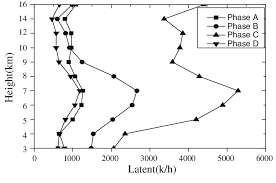The image above represents total latent heat.

To compute for total latent heat, three essential parameters are needed and these parameters are Density of Solidifying Metal (ρ’), Volume of Casting (V) and Latent Heat of Fusion (Hf).

The formula for calculating the total latent heat:

Q = ρ’VHf

Where:

Q = Total Latent Heat
ρ = Density of Solidifying Metal
V = Volume of Casting
Hf = Latent Heat of Fusion

Let’s solve an example;
Find the total latent heat when the density of solidifying metal is 12, the volume of casting is 14 and the latent heat of fusion is 10.

This implies that;

ρ = Density of Solidifying Metal = 12
V = Volume of Casting = 14
Hf = Latent Heat of Fusion = 10

Q = ρ’VHf
Q = (12)(14)(10)
Q = 1680

Therefore, the total latent heat is 1680 J/Kg.

Calculating the Density of Solidifying Metal when the Total Latent Heat, the Volume of Casting and the Latent Heat of Fusion is Given.

ρ = Q / VHf

Where;

ρ = Density of Solidifying Metal
Q = Total Latent Heat
V = Volume of Casting
Hf = Latent Heat of Fusion

Let’s solve an example;
Find the density of solidifying metal when the total latent heat is 20, the volume of casting is 4 and the latent heat of fusion is 2.

This implies that;

Q = Total Latent Heat = 20
V = Volume of Casting = 4
Hf = Latent Heat of Fusion = 2

ρ = Q / VHf
ρ = 20 / (4)(2)
ρ = 20 / 8
ρ = 2.5

Therefore, the density of solidifying metal is 2.5

Calculating the Volume of Casting when the Total Latent Heat, the Density of Solidifying Metal and the Latent Heat of Fusion is Given.

V = Q / ρHf

Where;

V = Volume of Casting
Q = Total Latent Heat
ρ = Density of Solidifying Metal
Hf = Latent Heat of Fusion

Let’s solve an example;
Find the volume of casting when the total latent heat is 18, the density of solidifying metal is 3 and the latent heat of fusion is 3.

This implies that;

Q = Total Latent Heat = 18
ρ = Density of Solidifying Metal = 3
Hf = Latent Heat of Fusion = 3

V = Q / ρHf
V = 18 / (3)(3)
V = 18 / 9
V = 2

Therefore, the volume of casting is 2.

Calculating the Latent Heat of Fusion when the Total Latent Heat, the Density of Solidifying Metal and the Volume of Casting is Given.

Hf = Q / ρV

Where;

Hf = Latent Heat of Fusion
Q = Total Latent Heat
ρ = Density of Solidifying Metal
V = Volume of Casting

Let’s solve an example;
Find the latent heat of fusion when the total latent heat is 44, the density of solidifying metal is 11 and the volume of casting is 2.

This implies that;

Q = Total Latent Heat = 44
ρ = Density of Solidifying Metal = 11
V = Volume of Casting = 2

Hf = Q / ρV
Hf = 44 / (11)(2)
Hf = 44 / 22
Hf = 2

Therefore, the latent heat of fusion is 2.

Nickzom Calculator – The Calculator Encyclopedia is capable of calculating the total latent heat.

To get the answer and workings of the total latent heat using the Nickzom Calculator – The Calculator Encyclopedia. First, you need to obtain the app.

You can get this app via any of these means:

To get access to the professional version via web, you need to register and subscribe for NGN 2,000 per annum to have utter access to all functionalities.
You can also try the demo version via https://www.nickzom.org/calculator

Apple (Paid) – https://itunes.apple.com/us/app/nickzom-calculator/id1331162702?mt=8
Once, you have obtained the calculator encyclopedia app, proceed to the Calculator Map, then click on Materials and Metallurgical under Engineering.Now, Click on Solidification of Metal under Materials and Metallurgical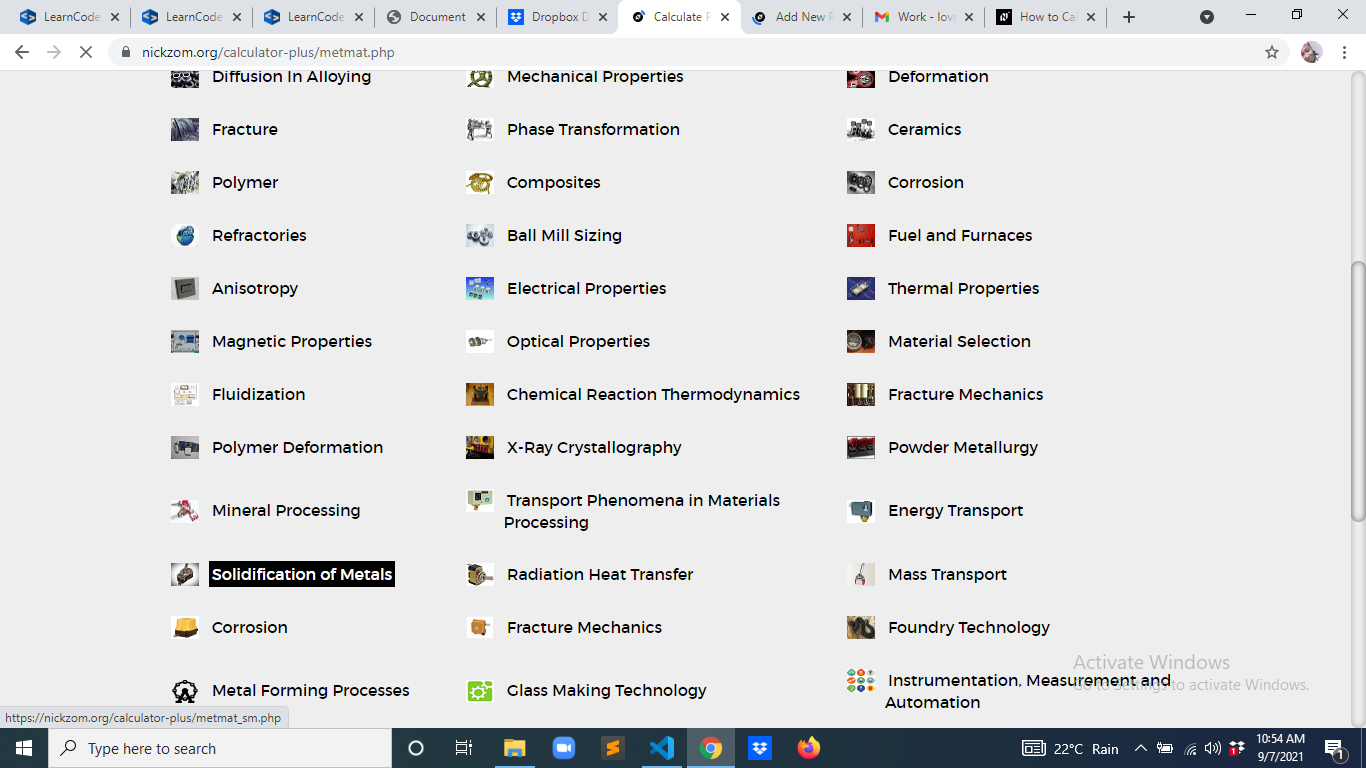Now, Click on Total Latent Heat under Solidification of Metal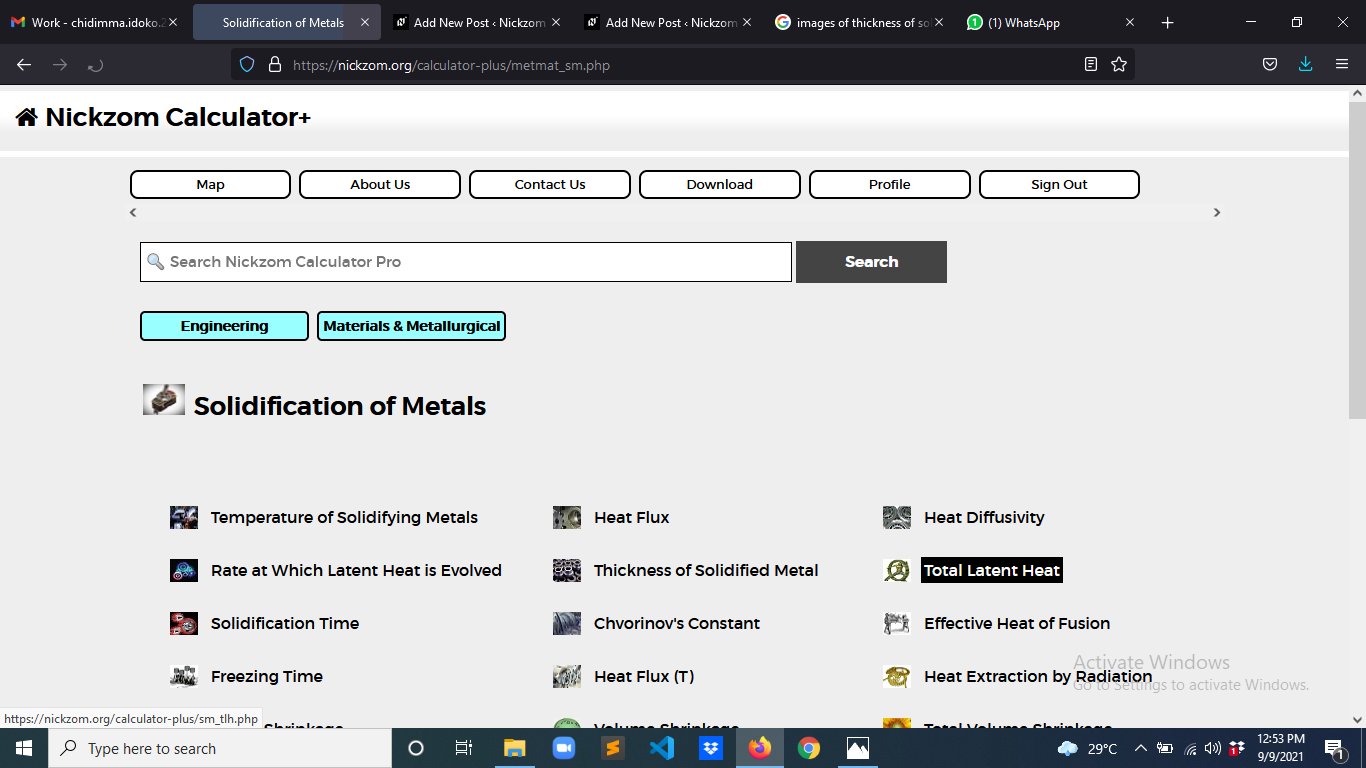The screenshot below displays the page or activity to enter your values, to get the answer for the total latent heat according to the respective parameter which is the Density of Solidifying Metal (ρ’), Volume of Casting (V) and Latent Heat of Fusion (Hf).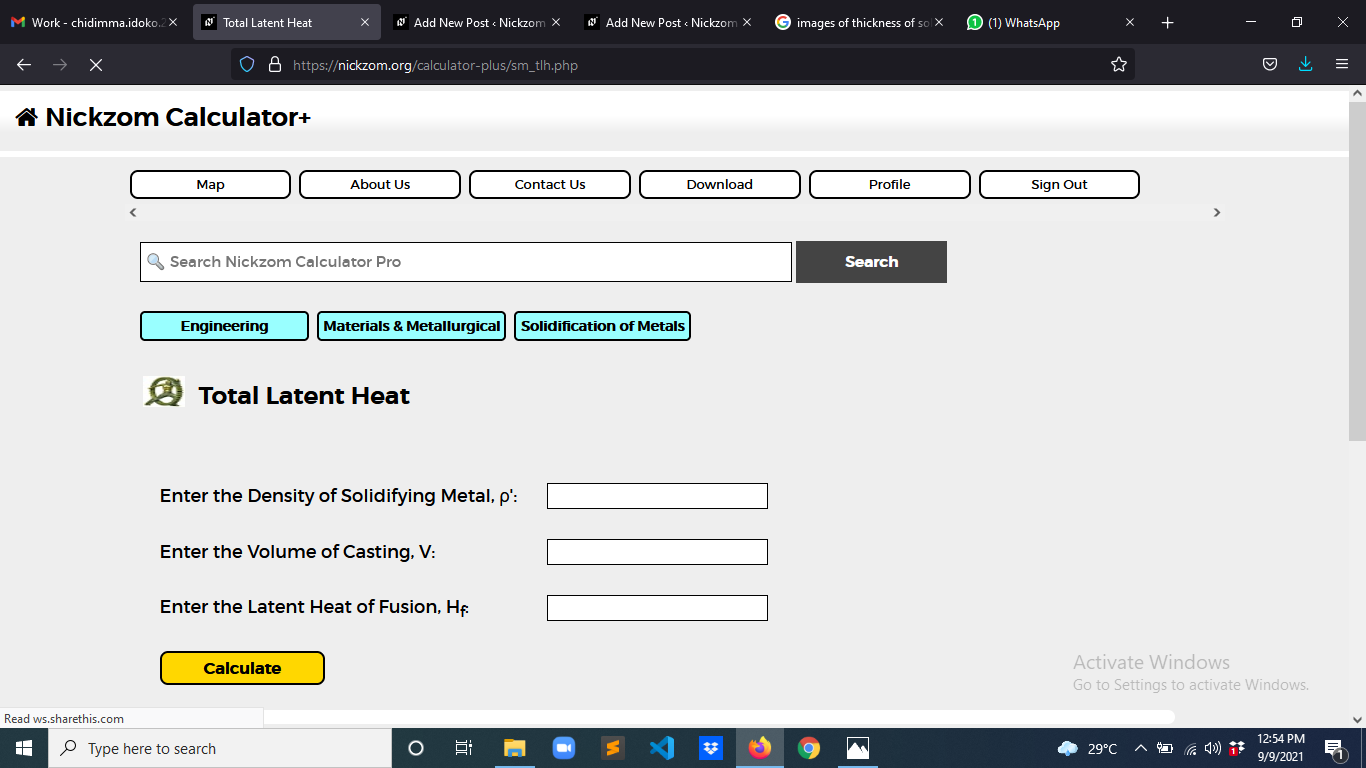Now, enter the values appropriately and accordingly for the parameters as required by the Density of Solidifying Metal (ρ’) is 12, Volume of Casting (V) is 14 and Latent Heat of Fusion (Hf) is 10.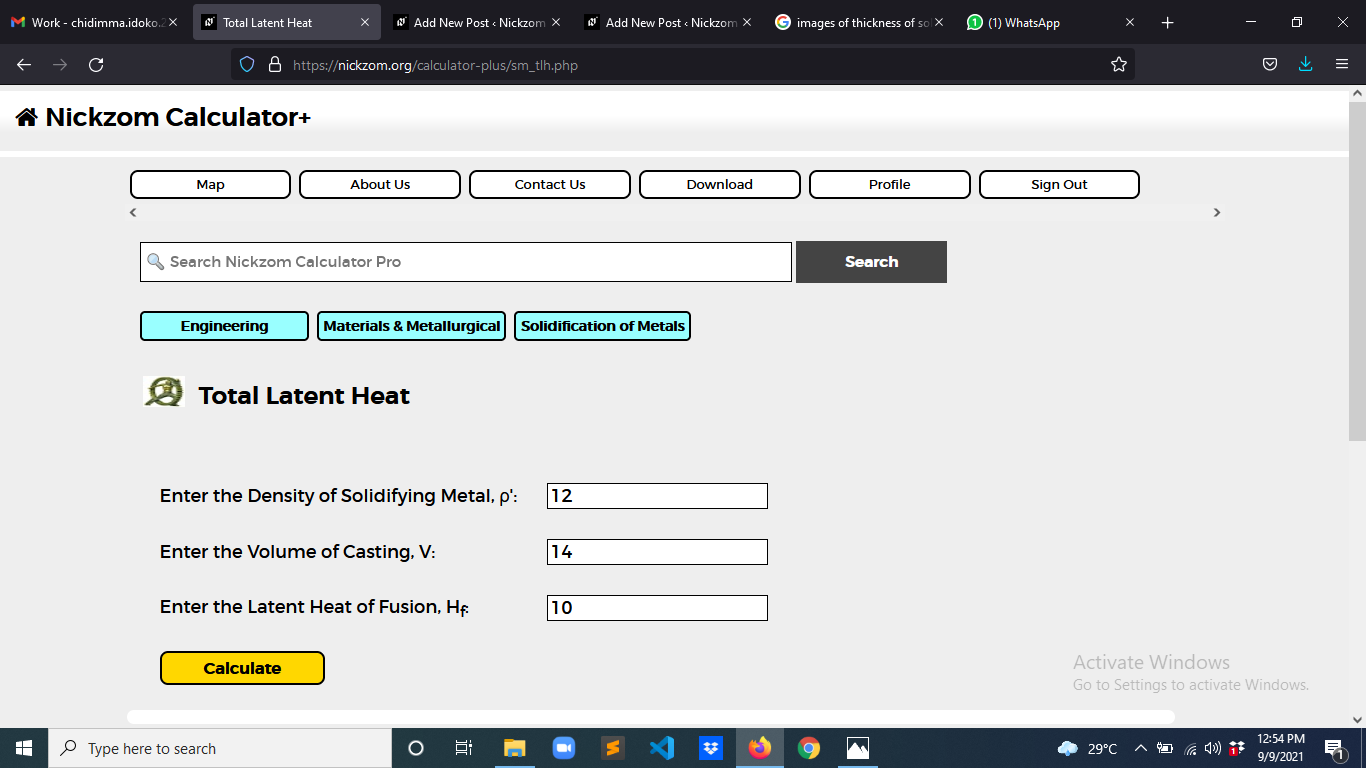Finally, Click on Calculate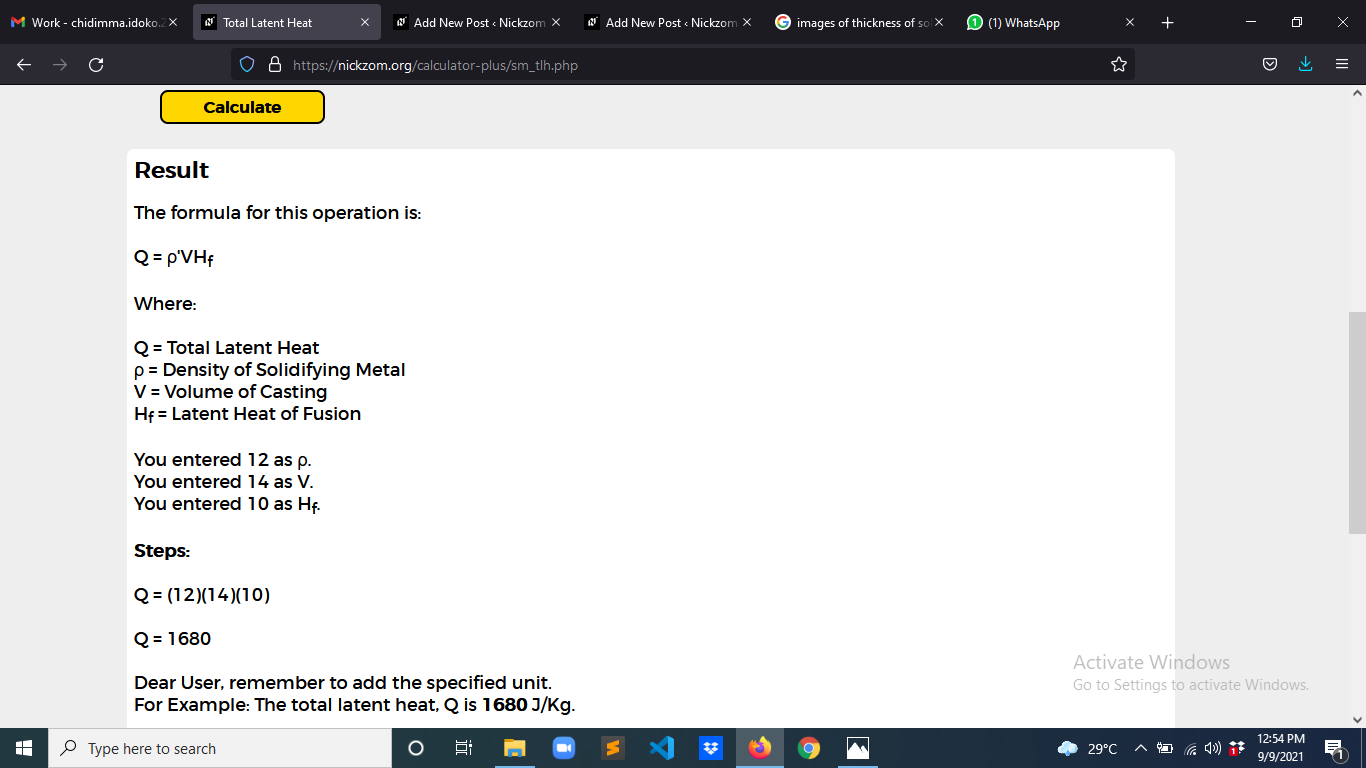As you can see from the screenshot above, Nickzom Calculator– The Calculator Encyclopedia solves for the total latent heat and presents the formula, workings and steps too.